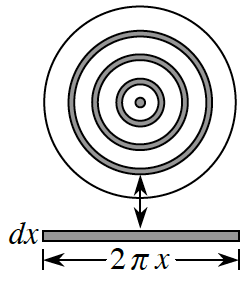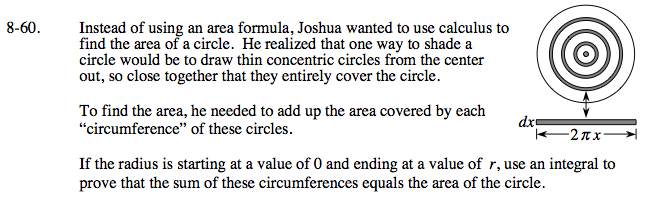### Home > CALC > Chapter 8 > Lesson 8.1.6 > Problem8-60

8-60.
1. Instead of using an area formula, Joshua wanted to use calculus to find the area of a circle. He realized that one way to shade a circle would be to draw thin concentric circles from thecenter out, so close together that they entirely cover the circle.

To find the area, he needed to add up the area covered by each "circumference" of these circles.

2. If the radius is starting at a value of 0 and ending at a value of r, use an integral to prove that the sum of these circumferences equals the area of the circle. Homework Help ✎Instead of integrating disks, imagine integrating rectangles (or infinitely skinny prisms) that wrap around a center point to create a solid.

Write an expression for the circumference in terms of r.